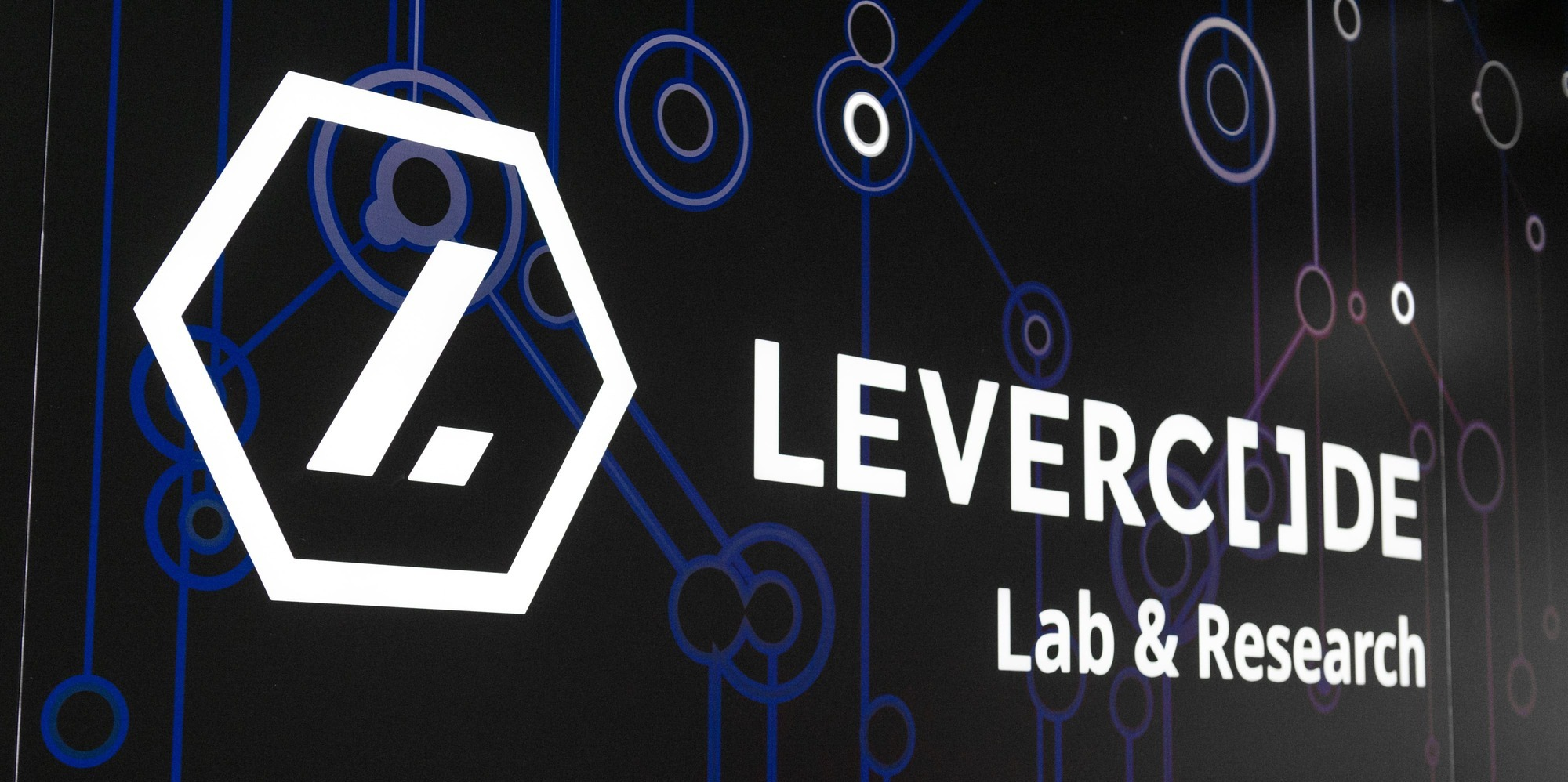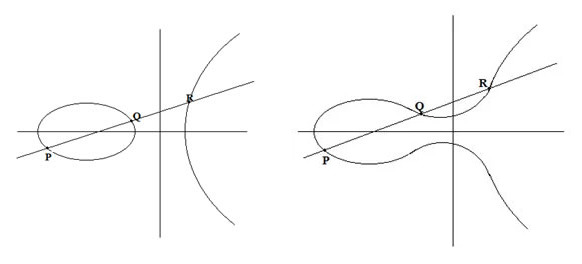20 June 2022

# It’s time to abandon ageing algorithms. The future is elliptic.

## What would be an alternative to ageing algorithms that are the foundation of many modern systems?

Even though elliptic-curve cryptography (ECC) has existed since the middle of the 1980s, it is still considered a newcomer in the world of SSL (Secure Sockets Layer). It has seen implementation only in the last few years.

If you’ve already heard something about ECC, you’ll probably know that it should be better than RSA. In order to take a dive into this topic, we must first clarify what the acronyms used in the world of cryptography mean.

RSA: Rivest–Shamir–Adleman (the three inventors of RSA)

RSA is old-school cryptography that uses prime factorisation. It dates back to the 1970s. RSA was the most popular method for encrypting the public key and for digital signing. Nowadays, Estonia uses elliptic cryptography.

ECC: elliptic-curve cryptography

This is newer cryptography that uses elliptic curves. This means more secure and shorter keys than with RSA.

ECDSA: elliptic curve digital signature algorithm

Logical explanation – this is an algorithm for digital signing that uses elliptic curves. Fortunately, the nature of digital signatures do not need to be explained to Estonians.

Edwards curve:

The Edwards curve is a special type of elliptic curve. The majority of elliptic curves are Montgomery curves (introduced by Peter L. Montgomery, a mathematician from the U.S.). Edwards curves were described by the mathematician Harold Edwards and made popular by the cryptographer Daniel Bernstein. They have a different structure which allows using EdDSA, a faster digital signing algorithm. The EdDSA algorithm works for a limited period of time, which means that it is faster and leaks less data.

In Estonia, Edwards elliptic curves are already used by LeverID, launched by the Estonian company Levercode. LeverID is a platform that is already in use and available for everyone. The mobile app can be downloaded from Google Play Store by everybody. The goal of LeverID is to cooperate with both start-ups and mature companies who wish to introduce the capacity of globally scalable and extremely secure authentication and digital signing to their services and customer interactions. We wrote in detail about LeverID in our blog in May.

## What is the difference between RSA and elliptic-curve cryptography?

When compared to the well-known RSA algorithm, elliptic-curve cryptography is an essentially different mathematical approach to encryption. Elliptic curve is the algebraic function (y2 = x3 + ax + b), which is drawn as a symmetric curve parallel to the x-axis (see drawings below).

Like other methods of public key cryptography, ECC is based on a unidirectional property, meaning that it is simple to make calculations, but the calculation results cannot be reversed in order to find the initial numbers. The difference is that ECC uses different mathematical operations than RSA in order to achieve this property.

Let’s try to explain this math as simply as possible. Let us use drawings to help us. The elliptic curve line passes through three points on the curve that are marked with the letters P, Q and R. If you know two points (P and Q), you can easily calculate the third one (R). However, if you only know R, there is no way to figure out the other two points, P and Q.The elliptic curve is an algebraic function. Photo: PKI Consortium

## Why is elliptic-curve cryptography better than RSA?

The main advantage of elliptic-curve cryptography lies in the fact that it is simply stronger than RSA for the key sizes currently used. A typical 256-bit ECC key is as secure as a 3,072-bit RSA key and 10,000 times stronger than a 2,048-bit RSA key.

In order to stay ahead of the calculation capacity of attackers, RSA keys need to become longer and longer. Leading browser makers announced already in 2013 that they would cease supporting 1,024-bit RSA keys. Therefore, all new SSL certificates must use keys that are twice as long. However, the issue with RSA is that the keys become exponentially bigger while the length of ECC keys increases on a linear basis together with strength.

The other security advantage of ECC is expressed in the fact that it simply offers an alternative to RSA and DSA (digital signature algorithm). When a severe weakness is discovered in RSA, ECC is most likely the best alternative, especially when compensating for the weakness in RSA requires an unexpected exponential increase in the key size.

Elliptic-curve cryptography is also faster for several reasons. Smaller keys mean that there is less data that must be forwarded to the client from the server in the course of operation. Additionally, ECC needs less processing capacity (CPU) and memory, resulting in a significantly faster response time and web server capacity when ECC is used.

In summary, it could be stated that ECC is a significant improvement of the cryptography used in SSL. It offers several advantages, including increased strength and capacity. Elliptic-curve cryptography offers a vital alternative to ageing algorithms that are still the foundation of many modern systems.